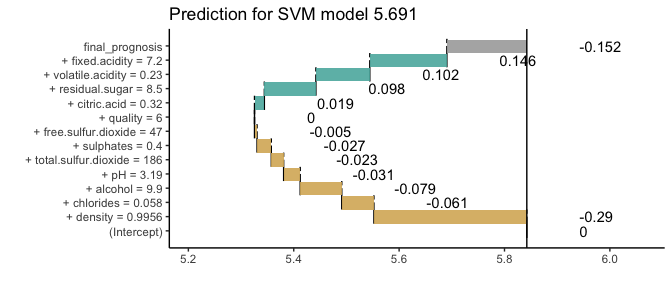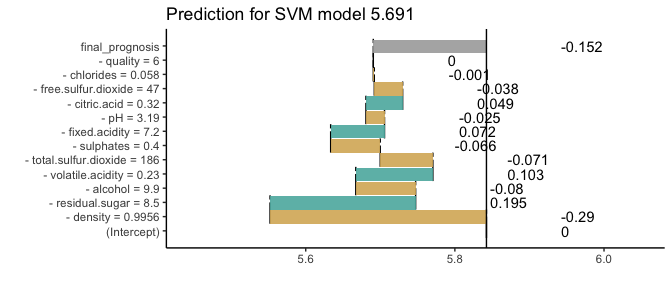# How to use breakDown package for SVM models

#### 2018-06-11

This example demonstrates how to use the breakDown package for models created with the kernlab package.

library("breakDown")
library(kernlab)
#>
#> Attaching package: 'kernlab'
#> The following object is masked from 'package:ggplot2':
#>
#>     alpha

wine_svm_model <- ksvm(quality~., data = wine)
wine_svm_model
#> Support Vector Machine object of class "ksvm"
#>
#> SV type: eps-svr  (regression)
#>  parameter : epsilon = 0.1  cost C = 1
#>
#> Gaussian Radial Basis kernel function.
#>  Hyperparameter : sigma =  0.0812662700662813
#>
#> Number of Support Vectors : 4196
#>
#> Objective Function Value : -2269.019
#> Training error : 0.522769

# or with the e1071:::svm

library(e1071)
wine_svm_model <- svm(quality~., data = wine)
wine_svm_model
#>
#> Call:
#> svm(formula = quality ~ ., data = wine)
#>
#>
#> Parameters:
#>    SVM-Type:  eps-regression
#>        cost:  1
#>       gamma:  0.09090909
#>     epsilon:  0.1
#>
#>
#> Number of Support Vectors:  4176

Now we are ready to call the broken() function. Since kernlab is useing S4 methods we need to pass here the hook to kernlab:::predict method.

library("breakDown")
nobs <- wine[5, , drop = FALSE]
base_prediction <- predict(wine_svm_model, nobs)
set.seed(1313)

explain_5_up <- broken(wine_svm_model, new_observation = nobs,
data = wine, predict.function = predict,
baseline = "intercept", direction = "up")
explain_5_up
#>                              contribution
#> (Intercept)                         0.000
#> + density = 0.9956                 -0.290
#> + chlorides = 0.058                -0.061
#> + alcohol = 9.9                    -0.079
#> + pH = 3.19                        -0.031
#> + total.sulfur.dioxide = 186       -0.023
#> + sulphates = 0.4                  -0.027
#> + free.sulfur.dioxide = 47         -0.005
#> + quality = 6                       0.000
#> + citric.acid = 0.32                0.019
#> + residual.sugar = 8.5              0.098
#> + volatile.acidity = 0.23           0.102
#> + fixed.acidity = 7.2               0.146
#> final_prognosis                    -0.152
#> baseline:  5.842347

explain_5_down <- broken(wine_svm_model, new_observation = nobs,
data = wine, predict.function = predict,
baseline = "intercept", direction = "down")
explain_5_down
#>                              contribution
#> (Intercept)                         0.000
#> - density = 0.9956                 -0.290
#> - residual.sugar = 8.5              0.195
#> - alcohol = 9.9                    -0.080
#> - volatile.acidity = 0.23           0.103
#> - total.sulfur.dioxide = 186       -0.071
#> - sulphates = 0.4                  -0.066
#> - fixed.acidity = 7.2               0.072
#> - pH = 3.19                        -0.025
#> - citric.acid = 0.32                0.049
#> - free.sulfur.dioxide = 47         -0.038
#> - chlorides = 0.058                -0.001
#> - quality = 6                       0.000
#> final_prognosis                    -0.152
#> baseline:  5.842347

And plot it.

library(ggplot2)
plot(explain_5_up) + ggtitle(paste0("Prediction for SVM model ", round(base_prediction, 3)))plot(explain_5_down) +  ggtitle(paste0("Prediction for SVM model ", round(base_prediction, 3)))